# Blurry Display STM32 with ILI9341

## Description

Blurry Display with Different Color

### What MCU/Processor/Board and compiler are you using?

STM32CubeIDE with GNU Compiler, I am Proteus Simulation Environment with STM32F401VE Microcontroller and ILI9341 connected with SPI Interface

Using LVGL

### What have you tried so far?

I wrote ILI9341 drivers and they seem to work also, but when integrated with LVGL I see a strange color display.

## Code to reproduce

Config file (also tried changing LV_COLOR_16_SWAP but didn’t help.

``````/*Color depth: 1 (1 byte per pixel), 8 (RGB332), 16 (RGB565), 32 (ARGB8888)*/

#define LV_COLOR_DEPTH     16

/*Swap the 2 bytes of RGB565 color. Useful if the display has a 8 bit interface (e.g. SPI)*/

#define LV_COLOR_16_SWAP   0

/*Enable more complex drawing routines to manage screens transparency.

*Can be used if the UI is above an other layer, e.g. an OSD menu or video player.

*Requires `LV_COLOR_DEPTH = 32` colors and the screen's `bg_opa` should be set to non LV_OPA_COVER value*/

#define LV_COLOR_SCREEN_TRANSP    0

/*Images pixels with this color will not be drawn if they are  chroma keyed)*/

#define LV_COLOR_CHROMA_KEY    lv_color_hex(0x00ff00)         /*pure green*/
``````

Below is the flush function. I think there is some problem with this function, which I copied from online, anyways I am trying to analyze but in case someone has some idea, please let me know.

``````static void tft_flush(lv_disp_drv_t * drv, const lv_area_t * area, lv_color_t * color_p)
{
uint32_t len = 0u;
lv_coord_t width = (area->x2 - area->x1) + 1;

/*Return if the area is out the screen*/
if(area->x2 < 0) return;
if(area->y2 < 0) return;
if(area->x1 > TFT_HOR_RES - 1) return;
if(area->y1 > TFT_VER_RES - 1) return;

/*Truncate the area to the screen*/
int32_t act_x1 = area->x1 < 0 ? 0 : area->x1;
int32_t act_y1 = area->y1 < 0 ? 0 : area->y1;
int32_t act_x2 = area->x2 > TFT_HOR_RES - 1 ? TFT_HOR_RES - 1 : area->x2;
int32_t act_y2 = area->y2 > TFT_VER_RES - 1 ? TFT_VER_RES - 1 : area->y2;

// Set Display Area
ILI9341_SetWindow( act_x1, act_y1, act_x2, act_y2 );
len = (act_x2 - act_x1 + 1u)*2u;
ILI9341_SendCommand(ILI9341_GRAM, 0u, 0u );

for( uint32_t y = act_y1; y <= act_y2; y++)
{
ILI9341_Send_16BitData( (uint16_t*)color_p, len );
color_p += width;
}
}
``````

And some ILI related function.

``````void ILI9341_SendCommand( uint8_t command, uint8_t *data, uint32_t length )
{
uint32_t timeout = length*10u;                    // Considering timeout as 10 * length milliseconds
CS_LOW();
DC_LOW();                                         // Command Mode
HAL_SPI_Transmit( &hspi2, &command, 1u, 10u );    // send command byte
DC_HIGH();                                        // Data Mode
if( length )
{
HAL_SPI_Transmit( &hspi2, data, length, timeout );
}
CS_HIGH();
}

void ILI9341_SendData( uint8_t *data, uint32_t length )
{
uint32_t timeout = length*10u;     // Considering timeout as 10 * length milliseconds
CS_LOW();
DC_HIGH();
HAL_SPI_Transmit( &hspi2, data, length, timeout );
CS_HIGH();
}

// Set the display area
void ILI9341_SetWindow( uint16_t x_start, uint16_t y_start, uint16_t x_end, uint16_t y_end )
{
uint8_t params = { 0 };

params = x_start >> 8u;
params = 0xFF & x_start;
params = x_end >> 8u;
params = 0xFF & x_end;
ILI9341_SendCommand( ILI9341_CASET, params, 4u );

params = y_start >> 8u;
params = 0xFF & y_start;
params = y_end >> 8u;
params = 0xFF & y_end;
ILI9341_SendCommand( ILI9341_RASET, params, 4u );
}

void ILI9341_Send_16BitData( uint16_t *data, uint32_t length )
{
uint32_t idx = 0u;
uint8_t value = { 0 };
CS_LOW();
DC_HIGH();
for( idx=0; idx<length; idx++ )
{
value = data[idx] >> 8u;
value = data[idx] && 0xFF;
HAL_SPI_Transmit( &hspi2, value, 2u, 10u );
}
CS_HIGH();
}
``````

## Screenshot and/or video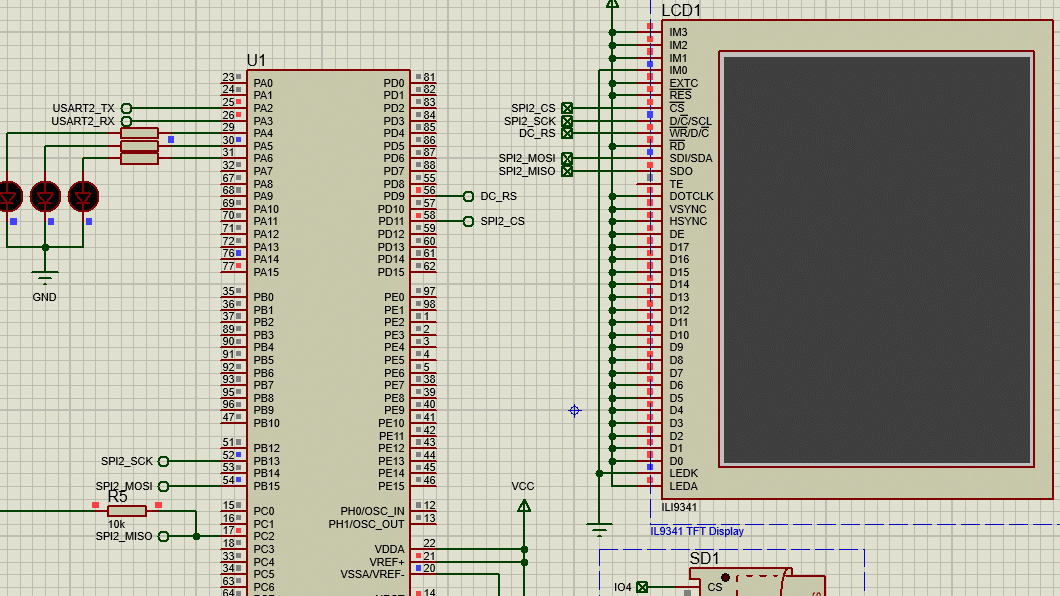I wanted to display a simple example of a label widget.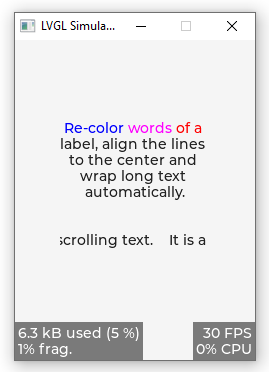This is the second post in this forum, and I didn’t get any response or suggestions.
I don’t the reason behind this, either my questions are really stupid or I am posting in the wrong section or etc.

The problem is still there, wanted to share one update which I tried.
`ILI9341_Send_16BitData` is a function I am only using inside the `tft_flush` function to send the data to the display.
Initially, I thought that there might be a problem with my `tft_flush` function, but then I changed my `ILI9341_Send_16BitData` function like below.

``````void ILI9341_Send_16BitData( uint16_t *data, uint32_t length )
{
uint32_t idx = 0u;
uint8_t value = { 0 };
CS_LOW();
DC_HIGH();
for( idx=0; idx<length; idx++ )
{
data[idx] = ILI9341_RED;    // --> this line added
value = data[idx] >> 8u;
value = data[idx] && 0xFF;
HAL_SPI_Transmit( &hspi2, value, 2u, 10u );
}
CS_HIGH();
}
``````

Here my intention was to ignore the data filled in buffers by LVGL, and instead of that just changing all pixel data with RED color, and this works, I can see RED color on the display.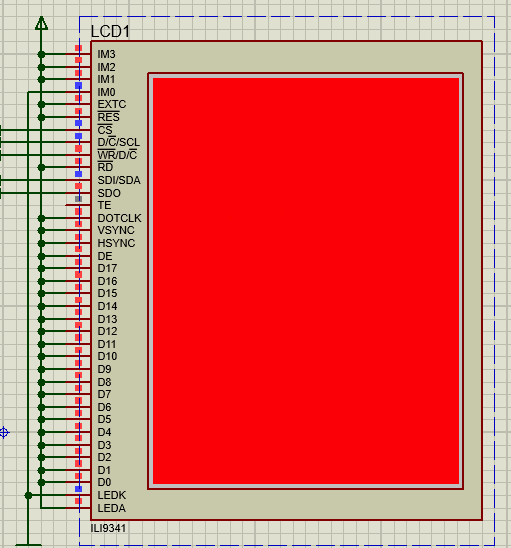I still doubt my `tft_flush` function and will keep on analyzing it, but I request from experts here support me with some hints to fix this issue.

Some updates from my side. I debugged and tried to first understand why the background is yellow.
So the issue is in the `ILI9341_Send_16BitData` function.
Instead of using the `AND` operator I used the `LOGICAL AND` and

`````` for( idx=0; idx<length; idx++ )
{
value = data[idx] >> 8u;
value = data[idx] & 0xFF;           // Earlier it was like value = data[idx] && 0xFF;
HAL_SPI_Transmit( &hspi2, value, 2u, 10u );
}
``````

And after fixing this I am getting the following on the screen.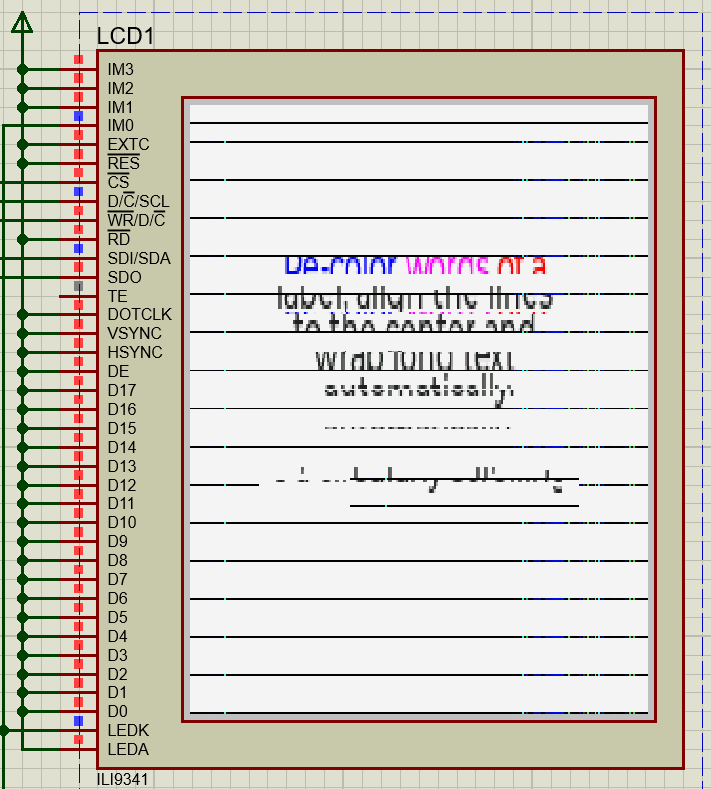Still not correct but I am investigating, but is 100% true that the problem is with my flush function.
I used the slow flush function and it works.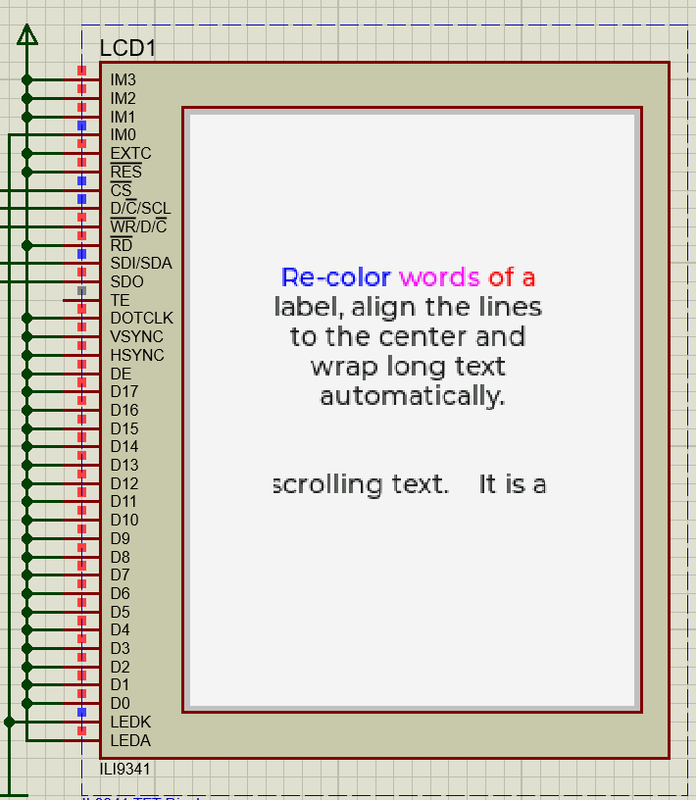Slow function is as below

``````static void tft_flush(lv_disp_drv_t * drv, const lv_area_t * area, lv_color_t * color_p)
{
uint16_t x, y;
uint16_t temp2 = 0;
lv_color_t temp;
temp = *color_p;
for(y = area->y1; y <= area->y2; y++)
{
for(x = area->x1; x <= area->x2; x++)
{
temp = *color_p;
temp2 = temp.full;
ILI9341_DrawPixel(x, y, temp2);
color_p++;
}
}

}
``````

Okay, I found the problem and fixed it, but still, there is another problem also.

``````//Flush Function
static void tft_flush(lv_disp_drv_t * drv, const lv_area_t * area, lv_color_t * color_p)
{
#define SPI_DATA_TRANSFER_8BIT        (0u)
#define SPI_DATA_TRANSFER_16BIT       (1u)
// #define SPI_DATA_TRANSFER_BITS        SPI_DATA_TRANSFER_8BIT
#define SPI_DATA_TRANSFER_BITS        SPI_DATA_TRANSFER_16BIT
uint16_t len = 0u;
lv_coord_t width = 0;

// return if area is out of screen
if(area->x2 < 0 || area->y2 < 0 || area->x1 > (TFT_HOR_RES  - 1) || area->y1 > (TFT_VER_RES  - 1))
{
return;
}

width = (area->x2 - area->x1) + 1;

// Truncate the area to the screen*
int32_t act_x1 = area->x1 < 0 ? 0 : area->x1;
int32_t act_y1 = area->y1 < 0 ? 0 : area->y1;
int32_t act_x2 = area->x2 > TFT_HOR_RES - 1 ? TFT_HOR_RES - 1 : area->x2;
int32_t act_y2 = area->y2 > TFT_VER_RES - 1 ? TFT_VER_RES - 1 : area->y2;

// calculate length of data to send over SPI (make sure to multiply by 2 if
// sending in 8-bit SPI transfer, and also set LV_COLOR_16_SWAP to 1
// for ex. RGB color is 0xF7BE, in 8 bit mode it will be sent as 0xBE & 0xF7
// hence set LV_COLOR_16_SWAP = 1 to swap this
#if (SPI_DATA_TRANSFER_BITS == SPI_DATA_TRANSFER_8BIT)
len = (act_x2 - act_x1 + 1u)*2u;
#elif (SPI_DATA_TRANSFER_BITS == SPI_DATA_TRANSFER_16BIT)
len = (act_x2 - act_x1 + 1u);
#endif

// Set Display Area
ILI9341_SetWindow( act_x1, act_y1, act_x2, act_y2 );

ILI9341_SendCommand(ILI9341_GRAM, 0u, 0u );

for( uint32_t y = act_y1; y <= act_y2; y++)
{
#if (SPI_DATA_TRANSFER_BITS == SPI_DATA_TRANSFER_8BIT)
ILI9341_SendData((uint8_t*)color_p, len);
#elif (SPI_DATA_TRANSFER_BITS == SPI_DATA_TRANSFER_16BIT)
ILI9341_Send_16BitData( (uint16_t*)color_p, len );
#endif
color_p += width;
}

}
``````

The above is the updated flush function, the problem is with the length when sending in 16-bit mode length should be.

``````len = (act_x2 - act_x1 + 1u);
``````

while in the 8-bit mode it should be

``````len = (act_x2 - act_x1 + 1u)*2;
``````

And I am using the 8-bit mode length in with a 16-bit function.
After fixing the results are as below.Another problem is with the 8-bit mode when I am updating the flush function like this.

``````  len = (act_x2 - act_x1 + 1u)*2;
for( uint32_t y = act_y1; y <= act_y2; y++)
{
ILI9341_SendData((uint8_t*)color_p, len);
color_p += width;
}
``````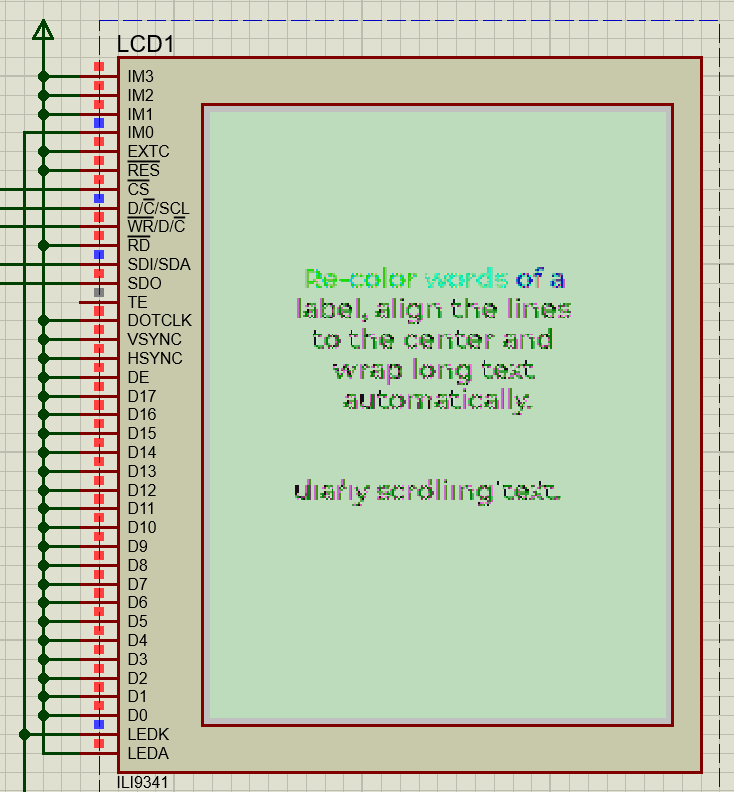Even after setting the macro to 1 doesn’t change anything.

``````#define LV_COLOR_16_SWAP   1
``````

So major issue is fixed, any suggestion here for this issue.